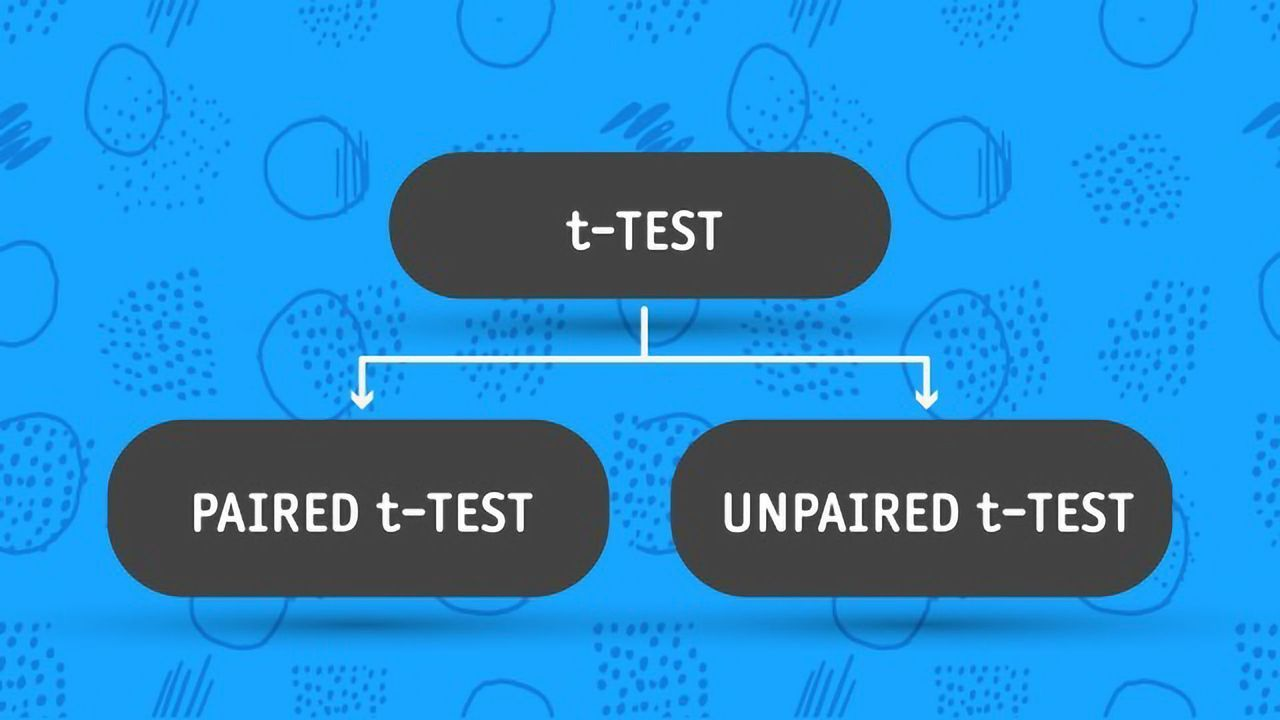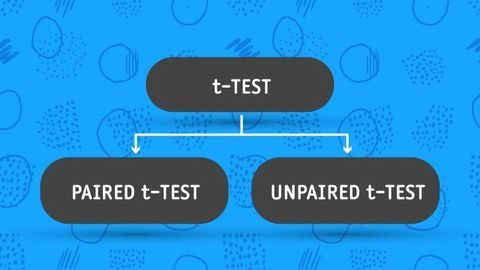We use cookies to provide you with a better experience. You can read our Cookie Policy here.Article

# Paired vs Unpaired T-Test: Differences, Assumptions and HypothesesArticle

# Paired vs Unpaired T-Test: Differences, Assumptions and Hypotheses

Complete the form below and we will email you a PDF version of "Paired vs Unpaired T-Test: Differences, Assumptions and Hypotheses"

First Name*
Last Name*
Country*
Company Type*
Job Function*
Would you like to receive further email communication from Technology Networks?

Technology Networks Ltd. needs the contact information you provide to us to contact you about our products and services. You may unsubscribe from these communications at any time. For information on how to unsubscribe, as well as our privacy practices and commitment to protecting your privacy, check out our Privacy Policy

Two-sample t-tests are statistical tests used to compare the means of two populations. Also known as Student’s t-tests, their results are used to determine if there is a significant difference between the mean of two samples that is unlikely to be due to sampling error or random chance.

Student’s t-tests are further broken down into two categories: paired t-tests and unpaired t-tests. These statistical tests are commonly used in research in the fields of biology, business, and psychology.

This article will explain when it is appropriate to use a paired t-test versus an unpaired t-test, as well as the hypothesis and assumptions of each.

## What is a paired t-test?

A paired t-test (also known as a dependent or correlated t-test) is a statistical test that compares the averages/means and standard deviations of two related groups to determine if there is a significant difference between the two groups.

●       A significant difference occurs when the differences between groups are unlikely to be due to sampling error or chance.

●       The groups can be related by being the same group of people, the same item, or being subjected to the same conditions.

Paired t-tests are considered more powerful than unpaired t-tests because using the same participants or item eliminates variation between the samples that could be caused by anything other than what’s being tested.

### What are the hypotheses of a paired t-test?

There are two possible hypotheses in a paired t-test.

●       The null hypothesis (H0) states that there is no significant difference between the means of the two groups.

●       The alternative hypothesis (H1) states that there is a significant difference between the two population means, and that this difference is unlikely to be caused by sampling error or chance.

### What are the assumptions of a paired t-test?

●       The dependent variable is normally distributed

●       The observations are sampled independently

●       The dependent variable is measured on an incremental level, such as ratios or intervals.

●       The independent variables must consist of two related groups or matched pairs.

## When to use a paired t-test?

Paired t-tests are used when the same item or group is tested twice, which is known as a repeated measures t-test. Some examples of instances for which a paired t-test is appropriate include:

●       The before and after effect of a pharmaceutical treatment on the same group of people.

●       Body temperature using two different thermometers on the same group of participants.

●       Standardized test results of a group of students before and after a study prep course.

## What is an unpaired t-test?

An unpaired t-test (also known as an independent t-test) is a statistical procedure that compares the averages/means of two independent or unrelated groups to determine if there is a significant difference between the two.

### What are the hypotheses of an unpaired t-test?

The hypotheses of an unpaired t-test are the same as those for a paired t-test. The two hypotheses are:

●       The null hypothesis (H0) states that there is no significant difference between the means of the two groups.

●       The alternative hypothesis (H1) states that there is a significant difference between the two population means, and that this difference is unlikely to be caused by sampling error or chance.

### What are the assumptions of an unpaired t-test?

●       The dependent variable is normally distributed

●       The observations are sampled independently

●       The dependent variable is measured on an incremental level, such as ratios or intervals.

●       The variance of data is the same between groups, meaning that they have the same standard deviation

●       The independent variables must consist of two independent groups.

## When to use an unpaired t-test?

An unpaired t-test is used to compare the mean between two independent groups. You use an unpaired t-test when you are comparing two separate groups with equal variance.

Examples of appropriate instances during which to use an unpaired t-test:

●       Research, such as a pharmaceutical study or other treatment plan, where ½ of the subjects are assigned to the treatment group and ½ of the subjects are randomly assigned to the control group.

●       Research during which there are two independent groups, such as women and men, that examines whether the average bone density is significantly different between the two groups.

●       Comparing the average commuting distance traveled by New York City and San Francisco residents using 1,000 randomly selected participants from each city.

In the case of unequal variances, a Welch’s test should be used.

## Paired vs unpaired t-test

The key differences between a paired and unpaired t-test are summarized below.

1. A paired t-test is designed to compare the means of the same group or item under two separate scenarios. An unpaired t-test compares the means of two independent or unrelated groups.
2. In an unpaired t-test, the variance between groups is assumed to be equal. In a paired t-test, the variance is not assumed to be equal.

## Paired vs unpaired t-test table